# Olympiad Test: Analytical Reasoning - 2

## 15 Questions MCQ Test Science Olympiad Class 8 | Olympiad Test: Analytical Reasoning - 2

Description
Attempt Olympiad Test: Analytical Reasoning - 2 | 15 questions in 30 minutes | Mock test for Class 8 preparation | Free important questions MCQ to study Science Olympiad Class 8 for Class 8 Exam | Download free PDF with solutions
QUESTION: 1

Solution:
QUESTION: 2

### In the following problems, find the number of parallelograms in each of the following figures.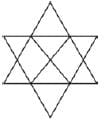Solution:

2 + 2 + 8 + 3 = 15.

QUESTION: 3

### In the following problems, find the number of parallelograms in each of the following figures.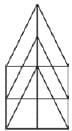Solution:
QUESTION: 4

In the following problems, find the number of parallelograms in each of the following figures.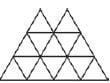Solution:
QUESTION: 5

In the following questions, what is the minimum number of straight lines ?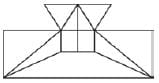Solution:
QUESTION: 6

In the following questions, what is the minimum number of straight lines ?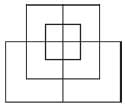Solution:
QUESTION: 7

In the following questions, what is the minimum number of straight lines ?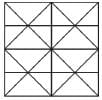Solution:
QUESTION: 8

In the following questions, what is the minimum number of straight lines ?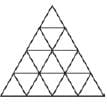Solution:
QUESTION: 9

In the following questions, what is the minimum number of straight lines ?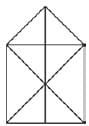Solution:
QUESTION: 10

In each of the following questions, what is the number of triangles and squares in the figure given below.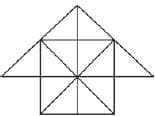Solution:
QUESTION: 11

In each of the following questions, what is the number of triangles and squares in the figure given below.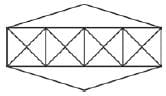Solution:
QUESTION: 12

In each of the following questions, what is the number of triangles and squares in the figure given below.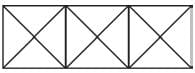Solution:
QUESTION: 13

In the following problems, find the number of parallelograms in each of thefollowing figures.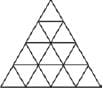Solution:
QUESTION: 14

In each of the following questions, what is the number of triangles and squares in the figure given below.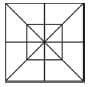Solution:
QUESTION: 15

In each of the following questions, what is the number of triangles and squares in the figure given below.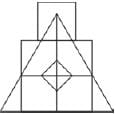Solution:Use Code STAYHOME200 and get INR 200 additional OFF Use Coupon Code